# Point of local extremum implies critical point

## Statement

Suppose$f$ is a function of one variable and$c$ is a point in the interior of the domain of$f$ (i.e.,$f$ is defined on an open interval containing$c$).

Suppose further that$c$ is a point of local extremum for$f$, i.e.,$f$ attains a local extreme value (either a local maximum or a local minimum) at$c$.

Then,$c$ is a critical point for$f$, i.e., either the derivative$\! f'(c)$ equals zero or the derivative$\! f'(c)$ does not exist.

Note that the$\! f'(c)$ not existing case could occur in either of these ways: one or both the one-sided derivatives of$f$ at$c$ not existing, or both one-sided derivatives of$f$ at$c$ existing but being unequal.

## Facts used

### Statement of facts

Fact no. (for reference in proof) Statement Assumption about one-sided local extremum Conclusion about sign of one-sided derivative Quick explanation in terms of the difference quotient$\frac{f(x) - f(c)}{x - c}$ Prototypical pictures
1 Local minimum from the left implies left hand derivative is nonpositive if it exists$f$ has a (possibly, but not necessarily, strict) local minimum from the left at$c$. The left hand derivative$f'_-(c)$, if it exists, is negative or zero In the difference quotient, the numerator is positive or zero and the denominator is negative. The quotient is thus negative or zero. Hence, so is the limit, if it exists.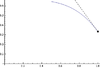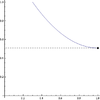2 Local maximum from the left implies left hand derivative is nonnegative if it exists (has full proof + video)$f$ has a (possibly, but not necessarily, strict) local maximum from the left at$c$. The left hand derivative$f'_-(c)$, if it exists, is positive or zero In the difference quotient, the numerator is negative or zero and the denominator is negative. The quotient is thus positive or zero. Hence, so is the limit, if it exists.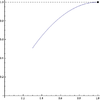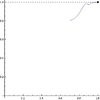3 Local minimum from the right implies right hand derivative is nonnegative if it exists$f$ has a (possibly, but not necessarily, strict) local minimum from the right at$c$. The right hand derivative$f'_+(c)$, if it exists, is positive or zero In the difference quotient, the numerator is positive or zero and the denominator is positive. The quotient is thus positive or zero. Hence, so is the limit, if it exists.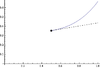4 Local maximum from the right implies right hand derivative is nonpositive if it exists$f$ has a (possibly, but not necessarily, strict) local maximum from the right at$c$. The right hand derivative$f'_+(c)$, if it exists, is negative or zero In the difference quotient, the numerator is negative or zero and the denominator is positive. The quotient is thus negative or zero. Hence, so is the limit, if it exists.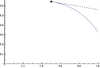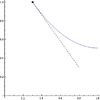The video below provides an intuitive explanation of the above facts. For a full proof, see the page on Fact (2).

### Note on strictness

All the facts as stated above are for not necessarily strict one-sided local extrema, i.e., we allow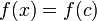$f(x) = f(c)$ for$x$ on the immediate left or right of$c$. However, even if we impose the additional condition of strictness, we still cannot eliminate the possibility of the one-sided derivative being zero. The reason is that even though the difference quotient must now be strictly positive or strictly negative in the various cases, the one-sided derivative, which is defined as a one-sided limit of the difference quotient, may still be equal to zero. All the prototypical pictures in the previous section are pictures of strict local extrema, and some of them show a one-sided derivative of zero.

For more, see the note on strictness in the proof for Fact (2).

This observation will be crucial when we piece together the two-sided information.

### Note on sign sensitivity

In the facts used above, we see that, when ascertaining the sign of the difference quotient$\frac{f(x) - f(c)}{x - c}$, the following are true:

• The sign of the numerator is governed by whether the point is a point of local maximum or minimum. For a point of local maximum, the numerator is negative or zero, and for a point of local minimum, the numerator is positive or zero.
• The sign of the denominator is governed by whether we are approaching from the left or the right. For a left-sided approach, the denominator is negative and for a right-sided approach, the denominator is positive.

The upshot of this is that if we change the direction of approach while preserving the nature of the local extreme value, the sign of the one-sided derivative flips. This is crucial to the proof.

## Proof

### Proof idea

The idea is to convert two-sided local extremum information into both its one-sided pieces, then determine the signs of the one-sided derivatives. As note in #Note on sign sensitivity, the sign conclusions for the two one-sided derivatives are opposite. We then pit these two pieces of information against each other to force the two-sided derivative, if it exists, to equal zero.

### Local minimum case

Given: A function$f$, a point$c$ in the interior of the domain of$f$ such that$f$ attains a local minimum at$c$, i.e.,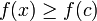$f(x) \ge f(c)$ for all$x \in (c - \delta, c + \delta)$ for some choice of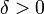$\delta > 0$.

To prove: If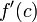$f'(c)$ exists, then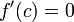$f'(c) = 0$

Proof

Step no. Assertion/construction Facts used Given data used Previous steps used Explanation
1 If the left hand derivative of$f$ at$c$ exists, then it is nonpositive, i.e., it is negative or zero. Fact (1)$f$ attains a local minimum at$c$ Since$f$ attains a two-sided local minimum at$c$, it in particular attains a local minimum from the left. Thus, Fact (1) applies.
2 If the right hand derivative of$f$ at$c$ exists, then it is nonnegative, i.e., it is positive or zero. Fact (3)$f$ attains a local minimum at$c$ Since$f$ attains a two-sided local minimum at$c$, it in particular attains a local minimum from the right. Thus, Fact (3) applies.
3 If the (two-sided) derivative of$f$ exists at$c$, it must be zero. Steps (1), (2) For the (two-sided) derivative to exist, both the left hand derivative and the right hand derivative must exist and they must be equal to each other and to the derivative. By Step (1), the derivative must be negative or zero. By Step (2), the derivative must be positive or zero. The only way both of these can be simultaneously true is if the derivative equals zero.

### Local maximum case

Given: A function$f$, a point$c$ in the interior of the domain of$f$ such that$f$ attains a local maximum at$c$, i.e.,$f(x) \le f(c)$ for all$x \in (c - \delta, c + \delta)$ for some choice of$\delta > 0$.

To prove: If$\! f'(c)$ exists, then$\!f'(c) = 0$

Proof

Step no. Assertion/construction Facts used Given data used Previous steps used Explanation
1 If the left hand derivative of$f$ at$c$ exists, then it is nonnegative, i.e., it is positive or zero. Fact (2)$f$ attains a local maximum at$c$ Since$f$ attains a two-sided local maximum at$c$, it in particular attains a local maximum from the left. Thus, Fact (2) applies.
2 If the right hand derivative of$f$ at$c$ exists, then it is nonpositive, i.e., it is negative or zero. Fact (4)$f$ attains a local maximum at$c$ Since$f$ attains a two-sided local maximum at$c$, it in particular attains a local maximum from the right. Thus, Fact (4) applies.
3 If the (two-sided) derivative of$f$ exists at$c$, it must be zero. Steps (1), (2) For the (two-sided) derivative to exist, both the left hand derivative and the right hand derivative must exist and they must be equal to each other and to the derivative. By Step (1), the derivative must be positive or zero. By Step (2), the derivative must be negative or zero. The only way both of these can be simultaneously true is if the derivative equals zero.